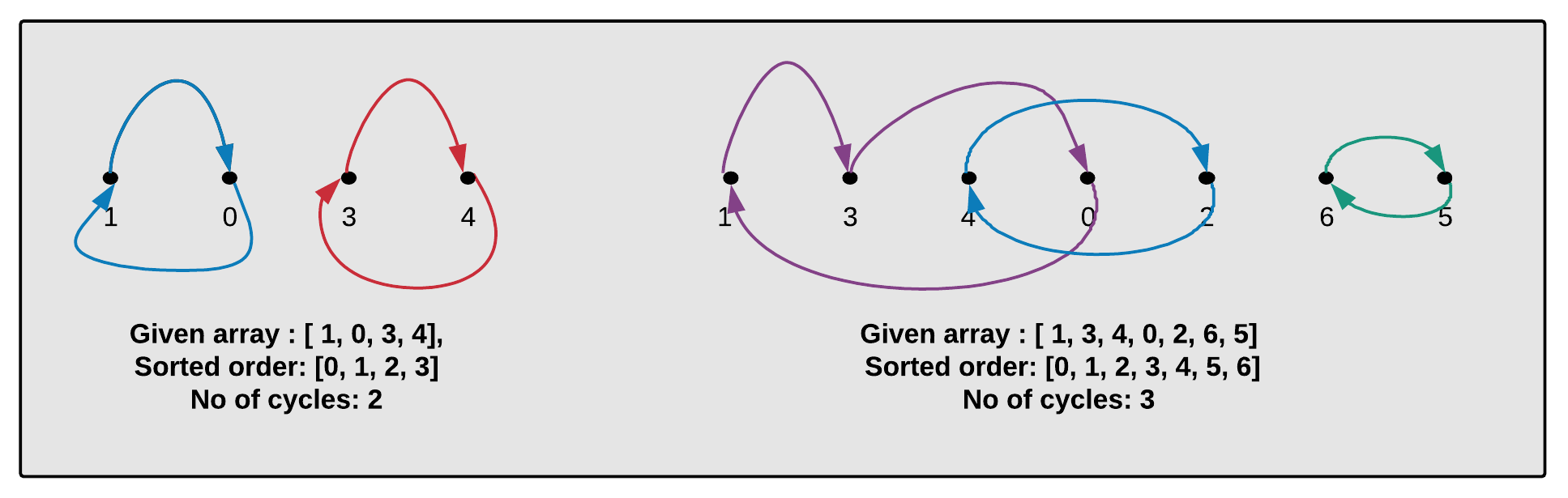Hard

# The number of cycles in a given array of integers.

Objective: Given an array of size N which contains integers from range 0 to N-1. (No duplicates). Write a program to find the number of cycles in the array.

Cycles in Array: Since the array is of size N and elements are from 0 to N-1 without any duplicates means all the elements appear exactly once. Create a graph with n vertices. Create an edge from vertex Vi to Vj if the element at position i should be in position j in the sorted ordering, With the logic above find the number of cycles in the given array. See the image below for better understanding.Example:

```Given Array : [3, 2, 1, 0]
Number of cycles: 2

Given Array : [2, 0, 1]
Number of cycles: 1

Given Array : [1, 3, 4, 0, 2, 6, 5]
Number of cycles: 3
```

Approach: Use DFS

1. Create a visited[] to check if the array element is already visited.
2. Initialize NoOfCycles = 0.
3. Now iterate through input[] created in step 1, for each index i
• If input[i] != i
• If i is not already visited then mark i as visited and jump to the next index which is at the index = input[i]. For example (1, 0) and i = 0 then jump to  index=1. Repeat this process until you find an index which is already visited.
• NoOfCycles++.
4. Return NoOfCycles.

Walk-through:walk-through:

```Input[] = [3, 2, 1, 0]
visited = [false, false, false, false]
NoOfCycles = 0

Index = 0, element = 3
0!=3 and 3 is not visited.
Mark 3 visited.
element 0 is not visited
Mark 0 visited.
NoOfCycles = 1
visited = [true, false, false, true]

Index = 1, process element = 2
1!=2 and 2 is not visited.
Mark 2 visited.
element 1 is not visited
Mark 1 visited.
NoOfCycles = 2
visited = [true, true, true, true]

NoOfCycles = 2
```

Output:

```Given Array : [3, 2, 1, 0] No of cycles: 2
Given Array : [2, 0, 1] No of cycles: 1
Given Array : [1, 3, 4, 0, 2, 6, 5] No of cycles: 3
```